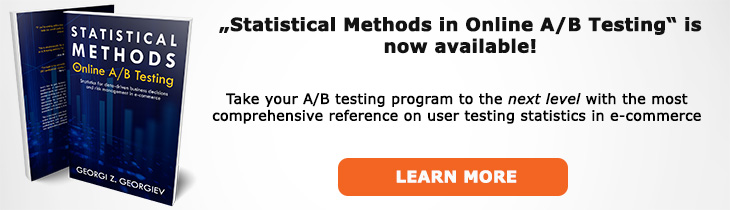# What does "Standard Error of the Mean" mean?

Definition of Standard Error of the Mean in the context of A/B testing (online controlled experiments).

## What is Standard Error of the Mean?

Aliases: SEM

The standard error of the mean of a distribution is the (population) standard deviation of the arithmetic mean, usually estimated through the sample standard deviation using the formula SEM = SDX / √N where N is the sample size. The standard error of the mean has a normal distribution regardless of the underlying distribution of the variable of interest (e.g. revenue per user, order value, session duration, page load speed) due to the Central Limit Theorem (CLT).

In A/B testing the standard error of the mean plays a central role since most of the statistics used are arithmetic averages. When reporting a confidence interval around a reported average one is just reporting the value that lies within x standard deviations of the mean so that 1-CDF(x) equals the confidence level. A p-value for an arithmetic mean is calculated in a similar manner by measuring the distance in standard errors between an observed mean and the hypothetical mean posited by the null hypothesis.

## Related A/B Testing terms

Standard Deviation

Like this glossary entry? For an in-depth and comprehensive reading on A/B testing stats, check out the book "Statistical Methods in Online A/B Testing" by the author of this glossary, Georgi Georgiev.## Glossary Index by Letter

Select a letter to see all A/B testing terms starting with that letter or visit the Glossary homepage to see all.